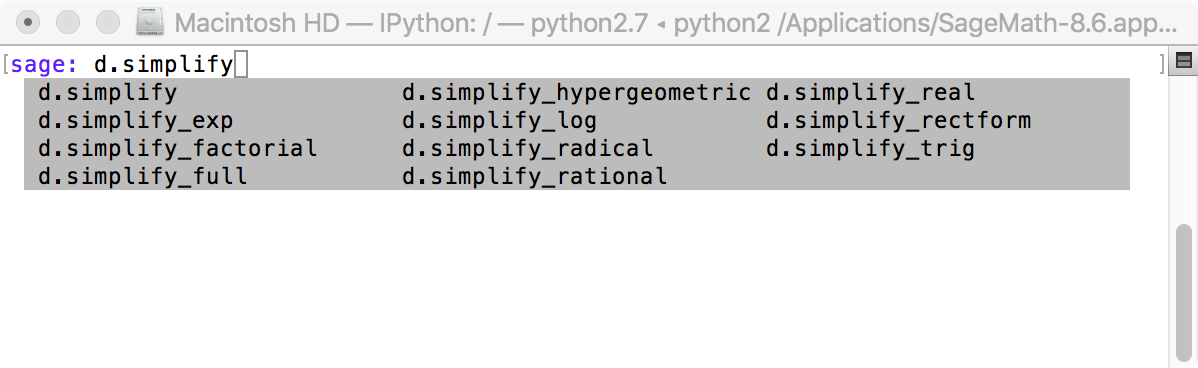# Best way to really simplify ugly formulas

As a casual user of sagemath I am always struggling to get my formulas simplified:

var('rg rs r');
dens = 1-r^2*rs/rg^3;
idens = 1-rs/rg;
ism = 1/4 *(3*sqrt(idens)-sqrt(dens))^2 * dens;
d = derivative(ism, r);
d.simplify_full();


The result has some potential for simplification, at least in terms of what think simplification might be. Can someone provide some hints as to how to get better results than from simplify_full()?

edit retag close merge delete

Sort by » oldest newest most votedIf you type

sage: d.simplify


and the tab key, you will see a pop-up frame showing different ways to complete that command to simplify d:Select one of them, say simplify_full, add ? and press the return key to see the associated help:

sage: d.simplify_full?
Docstring:
Apply "simplify_factorial()", "simplify_rectform()",
"simplify_trig()", "simplify_rational()", and then "expand_sum()"
to self (in that order).

ALIAS: "simplify_full" and "full_simplify" are the same.

EXAMPLES:

sage: f = sin(x)^2 + cos(x)^2
sage: f.simplify_full()
1

sage: f = sin(x/(x^2 + x))
sage: f.simplify_full()
sin(1/(x + 1))
...........................


In this case, since d contains radicals, you may try another method:

sage: d.canonicalize_radical()


which is the actual form of the deprecated simplify_radical. Check if the resulting simplification is good enough for you.

more